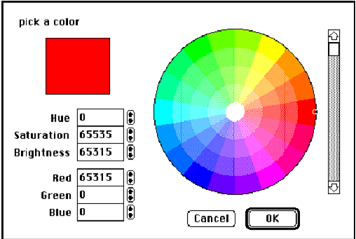## State cmy model, Data Structure & Algorithms

Assignment Help:

CMY Model

The cyan, magenta, yellow (CMY) colour model is a subtractive model based on the colour absorption properties of paints and inks. As such it has become the standard for many graphics output devices like ink jet and thermal transfer printers. The principle of the CMY model is in which white light beamed toward the viewer is intercepted by partially overlapping cyan, magenta, and yellow filters. The cyan filter removes red light from the beam and passes only cyan, the complementary colour to red.#### #title fcators of good algoritms, what are the factors for efficency of alg...

what are the factors for efficency of algoritms

#### Discuss the brute force algorithm, Question 1 What do you mean by Amortiza...

Question 1 What do you mean by Amortization? Question 2 Explain the following Big Oh notation (O) Omega notation (Ω) Theta notation (Θ)   Question 3 Di

#### Define ordinary variable, Ordinary variable An ordinary variable of a e...

Ordinary variable An ordinary variable of a easy data type can store a one element only

#### Complexity of an algorithm, An algorithm is a sequence of steps to solve a ...

An algorithm is a sequence of steps to solve a problem; there may be more than one algorithm to solve a problem. The choice of a particular algorithm depends upon following cons

#### State an algorithm which inputs 3 - digit code for 280 items, A small shop ...

A small shop sells 280 different items. Every item is identified by a 3 - digit code. All items which start with a zero (0) are cards, all items which start with a one (1) are swee

#### linear-expected-time algorithm, Implement a linear-expected-time algorithm...

Implement a linear-expected-time algorithm for selecting the k th smallest element Algorithm description 1. If |S| = 1, then k = 1 and return the element in S as the an

#### Program, What is a first-in-first-out data structure ? Write algorithms to...

What is a first-in-first-out data structure ? Write algorithms to perform the following operations on it – create, insertion, deletion, for testing overflow and empty conditions.

#### Surrounding of sub division method, Surrounding of sub division method ...

Surrounding of sub division method A polygon surrounds a viewport if it completely encloses or covers the viewport. This happens if none of its sides cuts any edge of the viewp

#### Spanning trees, Spanning Trees: A spanning tree of a graph, G, refer to a ...

Spanning Trees: A spanning tree of a graph, G, refer to a set of |V|-1 edges which connect all vertices of the graph. There are different representations of a graph. They are f

#### Efficient way of storing two symmetric matrices, Explain an efficient way o...

Explain an efficient way of storing two symmetric matrices of the same order in memory. A n-square matrix array is said to be symmetric if a[j][k]=a[k][j] for all j and k. For